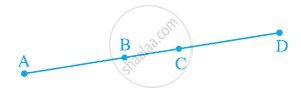# In the following figure, if AC = BD, then prove that AB = CD. - Mathematics

In the following figure, if AC = BD, then prove that AB = CD.#### Solution

From the figure, it can be observed that

AC = AB + BC

BD = BC + CD

It is given that AC = BD

AB + BC = BC + CD ......(1)

According to Euclid’s axiom, when equals are subtracted from equals, the remainders are also equal.

Subtracting BC from equation (1), we obtain

AB + BC − BC = BC + CD − BC

AB = CD

Concept: Euclid’S Definitions, Axioms and Postulates
Is there an error in this question or solution?
Chapter 5: Introduction to Euclid's Geometry - Exercise 5.1 [Page 86]

#### APPEARS IN

NCERT Class 9 Maths
Chapter 5 Introduction to Euclid's Geometry
Exercise 5.1 | Q 6 | Page 86

Share